Homework Help Question & Answers

# A) Derive the moment generating function (m.g.f) of the triangular distri- bution Y with p.d.f 0e...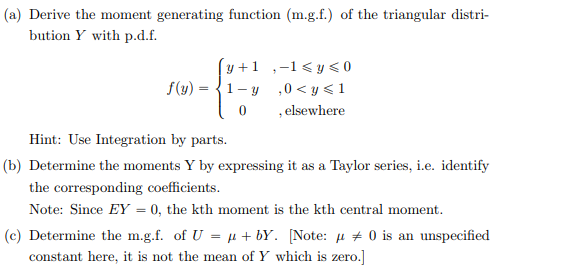Wanting help with part (b)

a) Derive the moment generating function (m.g.f) of the triangular distri- bution Y with p.d.f 0elsewhere Hint: Use Integration by parts. (b) Determine the moments Y by expressing it as a Taylor series, i.e. identify the corresponding coefficients Note: Since EY-0, the kth moment is the kth central moment (c) Determine the m.gf. of U-μ + bY. [Note: μ 0 is an unspecified constant here, it is not the mean of Y which is zero.]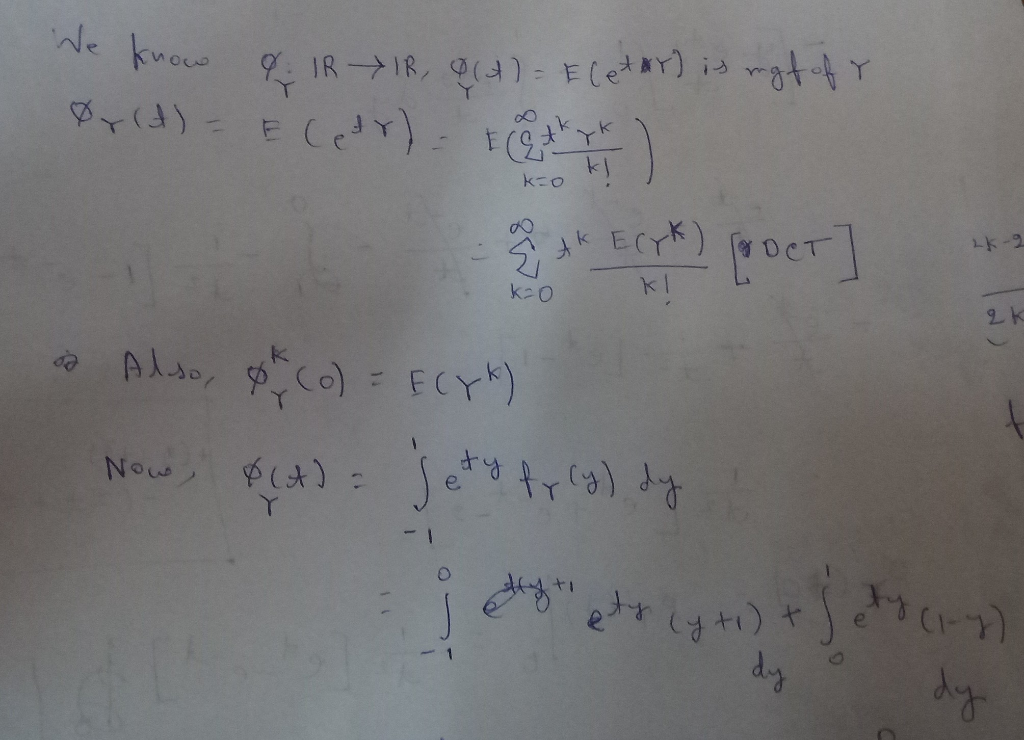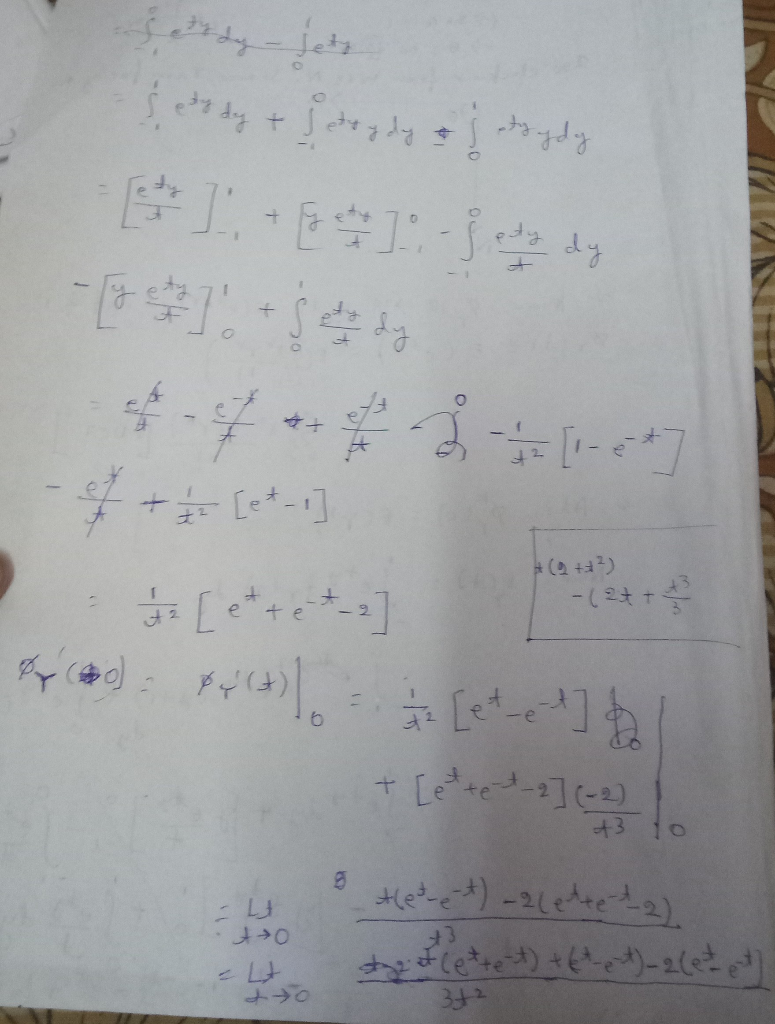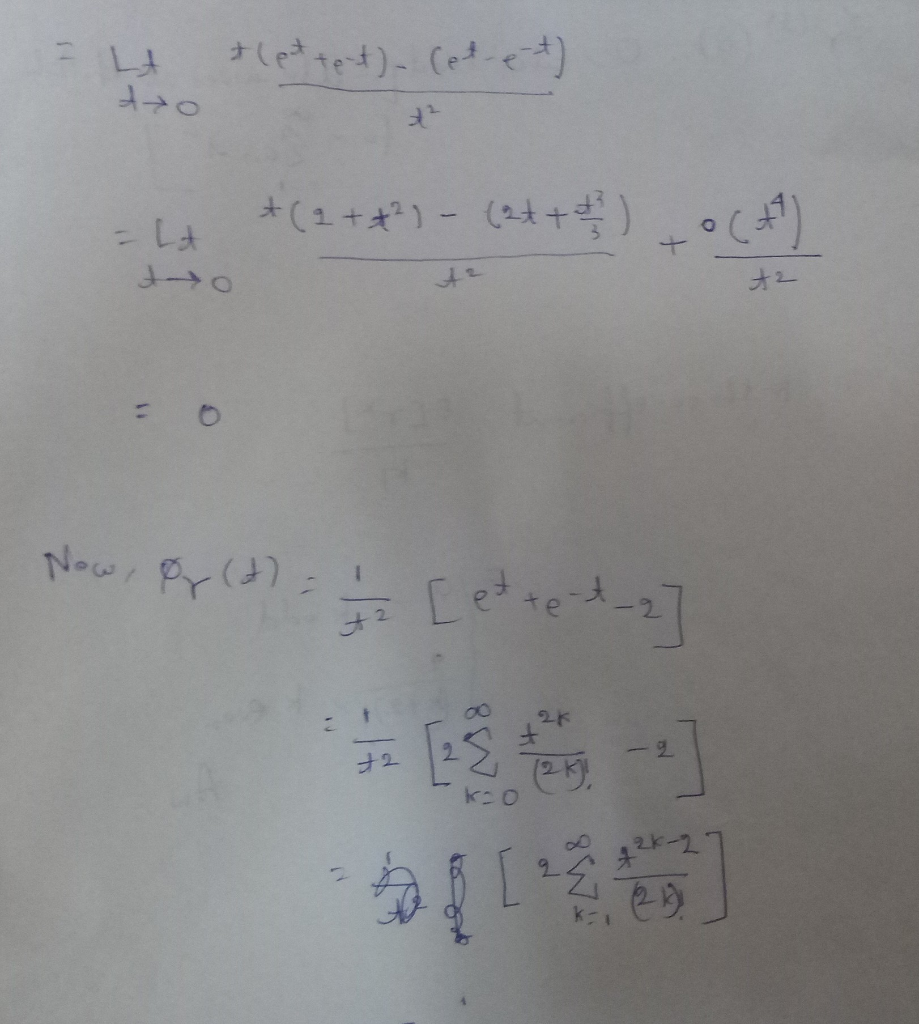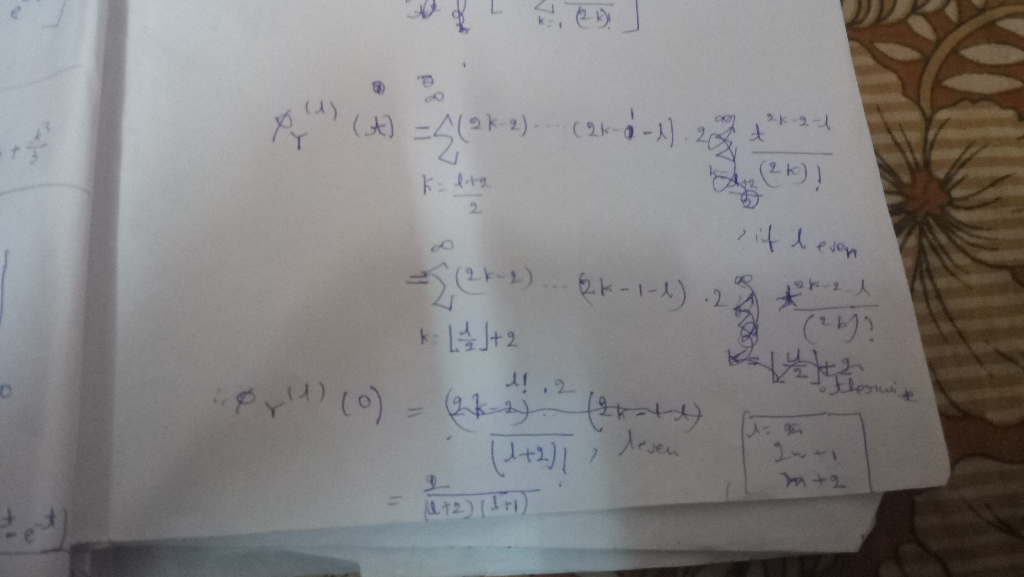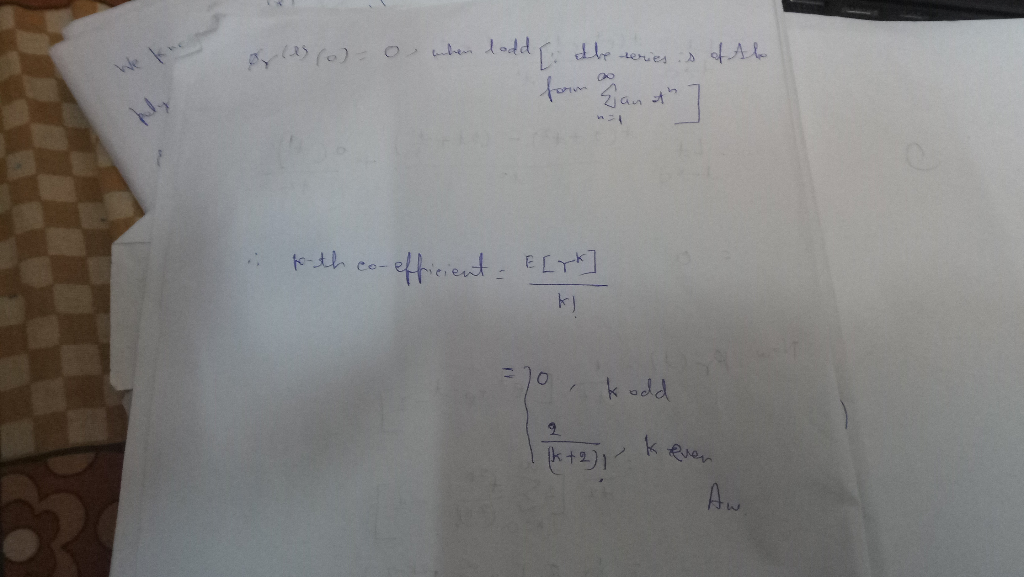##### Add Answer of: A) Derive the moment generating function (m.g.f) of the triangular distri- bution Y with p.d.f 0e...
More Homework Help Questions Additional questions in this topic.

• #### What is the difference between the shape of a side view of a triangular prism and a top view of a triangular prism

Need Online Homework Help?

Get FREE EXPERT Answers
WITHIN MINUTES
Related Questions